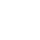Guangdong Kexin Engineering Management Co.,Ltd## 供水系统改造购置项目采购更正公告（第一次）

#### 二、更正信息：

 22 给水加压泵 Q=40m³/h，H=37m，P=5.5kw 台 6 23 给水加压泵 Q=24m³/h，H=38m，P=4kw 台 6 24 给水加压泵 Q=40m³/h，H=43m，P=7.5kw 台 4

 22 给水加压泵 Q=40m³/h，H=29m，P=5.5kw 台 9 23 给水加压泵 Q=24m³/h，H=38m，P=4kw 台 9 24 给水加压泵 Q=40m³/h，H=43m，P=7.5kw 台 6

 序号 加压设备参数 备注 1 Q=40m³/h，H=37m，P=5.5kw 所有给水加压泵均二用一备 2 Q=24m³/h，H=38m，P=4kw 3 Q=40m³/h，H=43m，P=7.5kw

 序号 加压设备参数 备注 1 Q=40m³/h，H=29m，P=5.5kw 所有给水加压泵均二用一备 2 Q=24m³/h，H=38m，P=4kw 3 Q=40m³/h，H=43m，P=7.5kw

#### 四、凡对本次公告内容提出询问，请按以下方式联系。

###### 3.项目联系方式

2021年11月02日

COPYRIGHT 广东科信工程管理有限公司  版权所有公安备案号：粤ICP备19051889号

• 取消“扫一扫”加入群聊